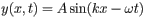# Problem: One formula for a wave with a y displacement (e.g., of astring) traveling in the x direction is.All the questions in this problem refer to this formula and to the wave it describes.a) What is the phase Φ(x t) of the wave?b) What is the wavelength λ of the wave?c) What is the period T of this wave?d) What is the speed of propagation v of this wave?

###### FREE Expert Solution

This problem requires us to analyze the description of waves using the given equation.

a)

The general equation of a wave is:

90% (4 ratings)###### Problem Details

One formula for a wave with a y displacement (e.g., of astring) traveling in the x direction is.

All the questions in this problem refer to this formula and to the wave it describes.

a) What is the phase Φ(x t) of the wave?

b) What is the wavelength λ of the wave?

c) What is the period T of this wave?

d) What is the speed of propagation v of this wave?